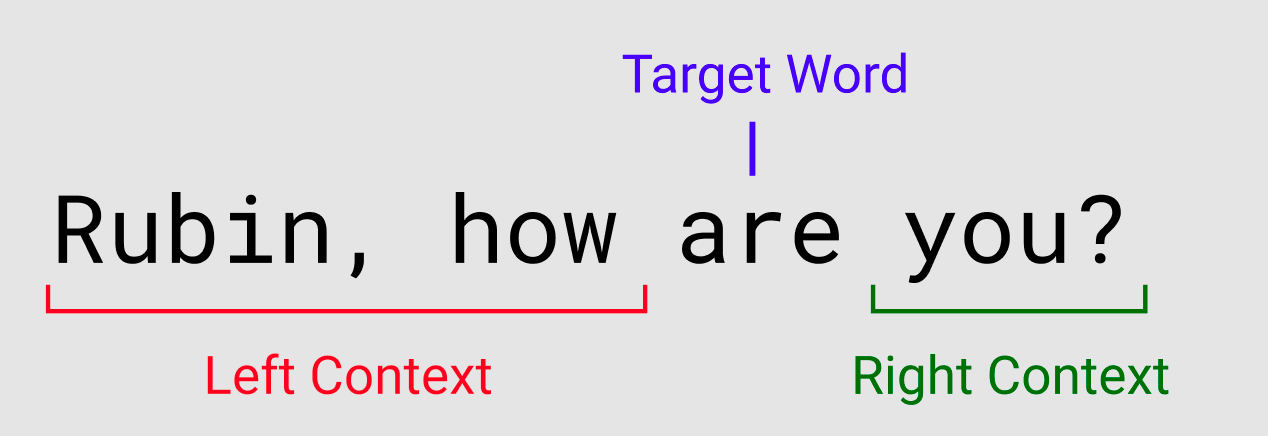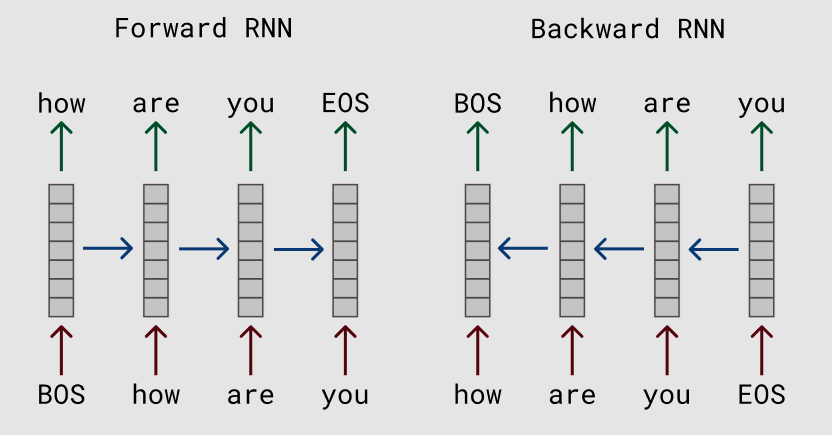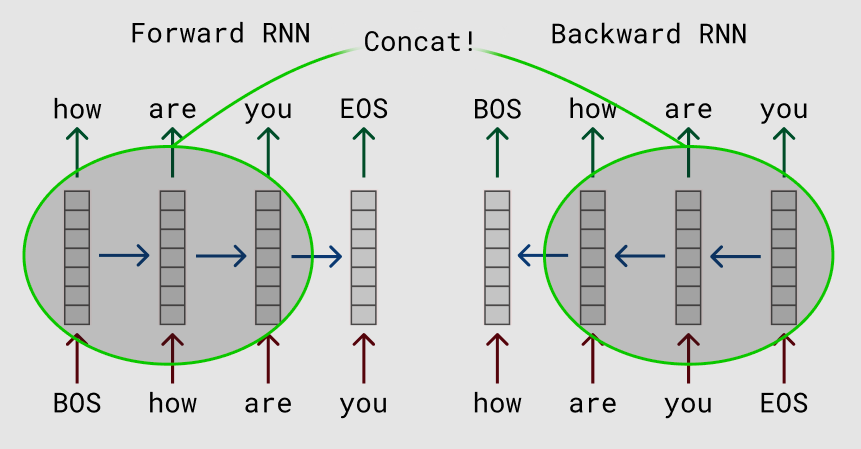# The Bidirectional Language Model

## Easy trick to include both left and right contexts

The usual approach in building a language model is to predict a word given the previous words. We can use either use an ngram language model or a variant of a recurrent neural network (RNN). An RNN (theoretically) gives us infinite left context (words to the left of the target word). But what we would really like is to use both left and right contexts see how well the word fits in the sentence. A bidirectional language model can enable this.

The problem statement: predict every word in the sentence given the rest of the words in the sentence.Predicting the word “are” from both left and right contexts.

Most deep learning frameworks will have support for bidirectional RNNs. They will usually return two sets of RNN hidden vectors where one is the output of the forward RNN and the other is the output of the backward RNN. These hidden vectors will be used to predict the next word in the sentence, where next word is the previous word for the backward RNN.Hidden vectors of the forward and backwarrd RNN.

Notice if we concatenated the two vectors at the same indices, the label will the input for the other RNN. For example at index 0, the forward RNN will predict on “how” but the backward RNN uses “how” as input. This results in a circular loop.

The simple trick is to stagger the hidden vectors so after concatenating them, they are predicting on the same token. Remember to add some padding at both ends of the sentence so we have enough context to predict the words. Here, we add a BOS (beginning of sentence) and EOS (end of sentence) padding tokens.Ignore the hidden vectors predicting the padding tokens and only focus on the vectors that predict on the words.

This is a trick that I’ve been using with success but haven’t seen too much in the literature much less the blogosphere (*ok found a few: here, here, here, here, here). Implementing this in your favorite neural network library should be fairly straightforward. Arguably, this trick with vanilla LSTMs will perform better than any careful finetuning of the unidirectional language model. The addition of the right context is very helpful.

Actually this method is similar to word2vec here you predict the target word given a moving window of left and right contexts. In our case with bidirectional language models, we have infinite context from the RNN.

The downside is, once you include the right context in a language model, you can’t use the model directly for language generation. But language generation is tricky and it’s the reason why chatbots are dead :P

Code in PyTorch below! Notice I stagger the bidirectional RNN hidden vectors by two (line 20).